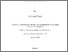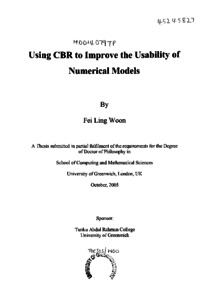# Using CBR to improve the usability of numerical models

Woon, Fei Ling (2005) Using CBR to improve the usability of numerical models. PhD thesis, University of Greenwich.Preview
PDF (Pages containing signatures redacted)
Fei Ling Woon 2005 - Redacted.pdf - Published Version

## Abstract

In this thesis we show that CBR systems can be constructed from numerical models, so as to improve their usability. It is shown that CBR models may be queried in a flexible manner, and that the user may formulate queries consisting of constraints over both “input” and “output” variables of the numerical model. It is also shown that the constraints may be formulated using either nominal or continuous variables. A generalization of the CBR retrieval process to include constraints over unified “input-output” space is formulated as a framework for the method.

The method is illustrated with practical engineering models: the pneumatic conveyor problem and the projectile problem. Comparisons are made on usability of CBR and numerical models for specific problems. It is shown that CBR models can answer questions difficult or impossible to formulate using numerical models, and that CBR models can be faster.

The thesis also addresses a latent problem with the general method, which is of importance generally. This is to do with interpolation over nominal values in unified space. A novel method is proposed for interpolation over nominal values, termed Generalised Shepard Nearest Neighbour method (GSNN). GSNN can utilise distance metrics defined on the solution space of a CBR system.

The properties and advantages of GSNN are examined in the thesis. A comparison is made with other CBR retrieval methods, using several examples, including the travel domain case base. It is shown that GSNN can out-perform conventional nearest neighbour methods. It is shown that GSNN has advantages in that it can find solutions not in the case base and it can find solutions not in the retrieval set. It is also shown that the performance of GSNN can be improved further by using it in conjunction with a diversity algorithm. The merit of using GSNN as a case selection component is examined, and it is shown that it can give good results in sparse case bases.

Finally the thesis concludes with a survey of numerical models where CBR construction can be useful, and where benefits can be expected.

Item Type: Thesis (PhD) uk.bl.ethos.437712 case-based reasoning, numerical analysis, numerical modelling, Q Science > QA Mathematics School of Computing & Mathematical SciencesSchool of Computing & Mathematical Sciences > Department of Computer Science 16 Feb 2017 17:06 http://gala.gre.ac.uk/id/eprint/6351View Item